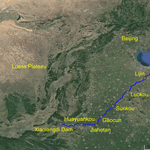https://doi.org/10.5194/esurf-6-989-2018
https://doi.org/10.5194/esurf-6-989-2018# Morphodynamic model of the lower Yellow River: flux or entrainment form for sediment mass conservation?

Chenge An, Andrew J. Moodie, Hongbo Ma, Xudong Fu, Yuanfeng Zhang, Kensuke Naito, and Gary Parker

Abstract. Sediment mass conservation is a key factor that constrains river morphodynamic processes. In most models of river morphodynamics, sediment mass conservation is described by the Exner equation, which may take various forms depending on the problem in question. One of the most widely used forms of the Exner equation is the flux-based formulation, in which the conservation of bed material is related to the stream-wise gradient of the sediment transport rate. An alternative form of the Exner equation, however, is the entrainment-based formulation, in which the conservation of bed material is related to the difference between the entrainment rate of bed sediment into suspension and the deposition rate of suspended sediment onto the bed. Here we represent the flux form in terms of the local capacity sediment transport rate and the entrainment form in terms of the local capacity entrainment rate. In the flux form, sediment transport is a function of local hydraulic conditions. However, the entrainment form does not require this constraint: only the rate of entrainment into suspension is in local equilibrium with hydraulic conditions, and the sediment transport rate itself may lag in space and time behind the changing flow conditions. In modeling the fine-grained lower Yellow River, it is usual to treat sediment conservation in terms of an entrainment (nonequilibrium) form rather than a flux (equilibrium) form, in consideration of the condition that fine-grained sediment may be entrained at one place but deposited only at some distant location downstream. However, the differences in prediction between the two formulations have not been comprehensively studied to date. Here we study this problem by comparing the results predicted by both the flux form and the entrainment form of the Exner equation under conditions simplified from the lower Yellow River (i.e., a significant reduction of sediment supply after the closure of the Xiaolangdi Dam). We use a one-dimensional morphodynamic model and sediment transport equations specifically adapted for the lower Yellow River. We find that in a treatment of a 200 km reach using a single characteristic bed sediment size, there is little difference between the two forms since the corresponding adaptation length is relatively small. However, a consideration of sediment mixtures shows that the two forms give very different patterns of grain sorting: clear kinematic waves occur in the flux form but are diffused out in the entrainment form. Both numerical simulation and mathematical analysis show that the morphodynamic processes predicted by the entrainment form are sensitive to sediment fall velocity. We suggest that the entrainment form of the Exner equation might be required when the sorting process of fine-grained sediment is studied, especially when considering relatively short timescales.

Share